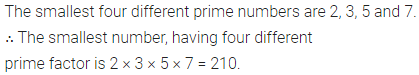# ML Aggarwal Class 6 Solutions for ICSE Maths Chapter 4 Playing with Numbers Ex 4.3

## ML Aggarwal Class 6 Solutions for ICSE Maths Chapter 4 Playing with Numbers Ex 4.3

Question 1.
Here are two different factor trees of the number 90. Write the missing numbers: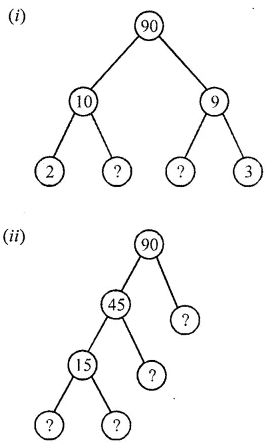Solution: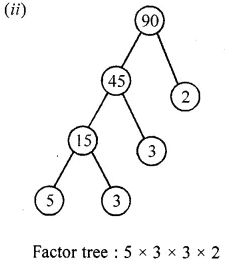Question 2.
Find the prime factorisation of the following numbers:
(i) 72
(ii) 172
(in) 450
(iv) 980
(v) 8712
(vi) 13500
Solution: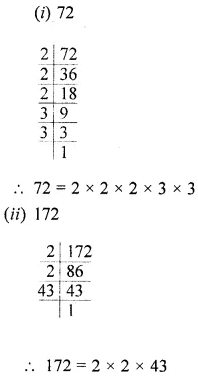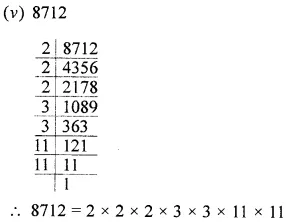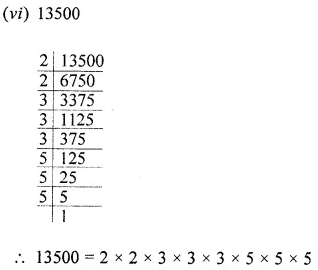Question 3.
Write the smallest and the greatest 3-digit numbers and express them as the product of prime.
Solution:Question 4.
Write the smallest five digit number and express it in the form of its prime factors.
Solution: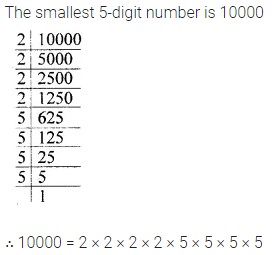Question 5.
1 am the smallest number, having four different prime factors. Can you find me?
Solution: